Next: norm Up: Attenuation of the noise Previous: Attenuation of the noise

## Preconditioning for accelerated convergence

A generally available preconditioning method is to change variables so that the regularization operator becomes an identity matrix Claerbout and Fomel (2002). The gradient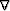in equation () has no inverse, but its spectrum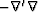, which appears in equation (), can be factored () into triangular partsand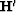whereis known as the Helix derivative and ()' denotes the adjoint. Thisis invertible by deconvolution Claerbout (1998). The fitting goals in equation () can be then rewritten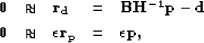(37)
with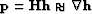and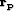is the residual for the new variable. I then minimize the misfit function(38)
and finally compute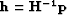to estimate the interpolated map of the lake. Experience shows that iterative solution forconverges much more rapidly than iterative solution for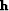thus showing thatis a good choice for preconditioning. There is no simple way of knowing beforehand what is the best value of. Practitioners like to see solutions for various values ofwhich can be computationally expensive. Practical exploratory data analysis is more pragmatic: without a simple clear theoretical basis, analysts generally begin from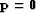and then abandon the fitting goal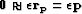Crawley (2000); Rickett et al. (2001); Trad et al. (2003). Implicitly, they take. Then they examine the solution as a function of iteration, imagining that the solution at larger iterations corresponds to smaller, and that the solution at smaller iterations corresponds to larger. In all my computations, I follow this approach and omit the regularization in the estimation of the depth maps.Next: norm Up: Attenuation of the noise Previous: Attenuation of the noise
Stanford Exploration Project
5/5/2005